Dalitz plot

Also found in: Wikipedia.

Dalitz plot

Pictorial representation in high-energy nuclear physics for data on the distribution of certain three-particle configurations. Many elementary-particle decay processes and high-energy nuclear reactions lead to final states consisting of three particles (which may be denoted by a, b, c, with mass values ma, mb, mc). Well-known examples are provided by the K-meson decay processes, Eqs. (1) and (2), and by the K- and -meson reactions with hydrogen, Eqs. (3) and (4).

(1)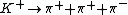(2)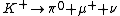(3)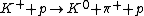(4)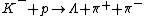For definite total energy E (measured in the barycentric frame), these final states have a continuous distribution of configurations, each specified by the way this energy E is shared among the three particles. (The barycentric frame is the reference frame in which the observer finds zero for the vector sum of the momenta of all the particles of the system considered.) See Elementary particle

If the three particles have kinetic energies Ta, Tb, and Tc (in the barycentric frame), Eq.

(5)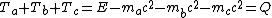(5) is obtained. As shown in the illustration, this energy sharing may be represented uniquely by a point F within an equilateral triangle LMN of side 2Q/3, such that the perpendiculars FA, FB, and FC to its sides are equal in magnitude to the kinetic energies Ta, Tb, and Tc. The most important property of this representation is that the area occupied within this triangle by any set of configurations is directly proportional to their volume in phase space.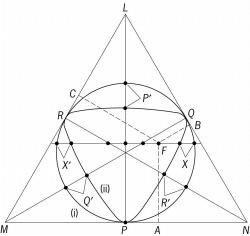Configuration of a three-particle system (abc) in its barycentric frame is specified by a point F such that the three perpendiculars FA, FB, and FC to the sides of an equilateral triangle LMN (of height Q) are equal in magnitude to the kinetic energies Ta, Tb, Tc, where Q denotes their sum

Not all points F within the triangle LMN correspond to configurations realizable physically, since the a, b, c energies must be consistent with zero total momentum for the three-particle system. With nonrelativistic kinematics and with equal masses m for a, b, c, the only allowed configurations are those corresponding to points F lying within the circle inscribed within the triangle, shown as (i) in the illustration. More generally, with relativistic kinematics, the limiting boundary is distorted as illustrated by the boundary curve (ii), drawn for the ω → 3π decay process, where the final masses are equal.

McGraw-Hill Concise Encyclopedia of Physics. © 2002 by The McGraw-Hill Companies, Inc.

Dalitz plot

[′dä·lits ‚plät]
(particle physics)
Pictorial representation for data on the distribution of certain three-particle configurations that result from elementary-particle decay processes or high-energy nuclear reactions.
McGraw-Hill Dictionary of Scientific & Technical Terms, 6E, Copyright © 2003 by The McGraw-Hill Companies, Inc.
References in periodicals archive ?
A long time ago, the D [right arrow] K[pi][pi] decay was studied with the Dalitz plot analysis .
In this work, we have calculated the branching ratios of the [B.sup.0] [right arrow] J/[psi][eta][K.sup.0] and [B.sup.+] [right arrow] J/[psi][eta][K.sup.+] decay by using the Dalitz plot analysis.
Site: Follow: Share:
Open / Close